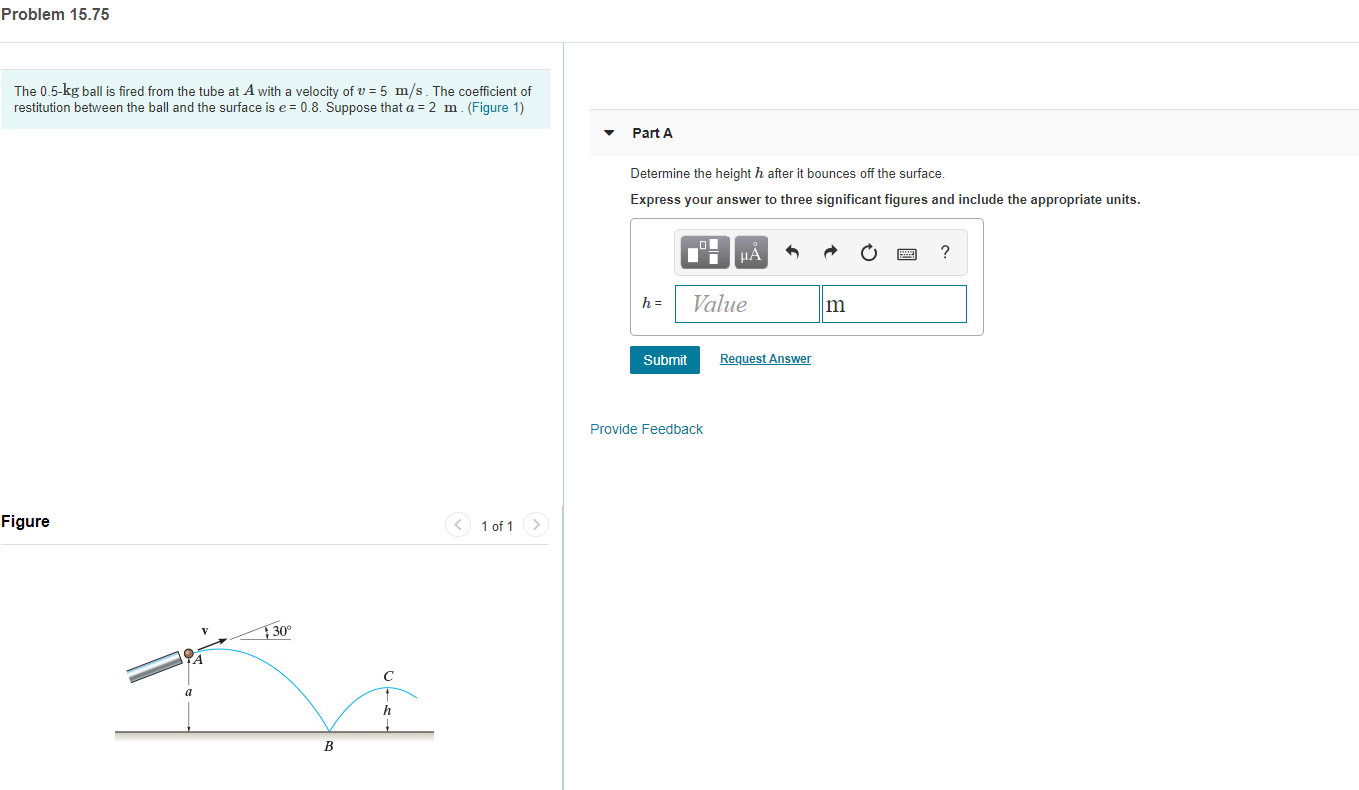# Problem 15.75The 0.5-kg ball is fired from the tube at A with a velocity of v 5 m/s The coefficient ofrestitution between the ball and the surface is e 0.8. Suppose that a = 2 m. (Figure 1)Part ADetermine the height h after it bounces off the surface.Express your answer to three significant figures and include the appropriate units.п‑НАValueh =mRequest AnswerSubmitProvide FeedbackFigure1 of 1>30аВ

Question
80 viewshelp_outlineImage TranscriptioncloseProblem 15.75 The 0.5-kg ball is fired from the tube at A with a velocity of v 5 m/s The coefficient of restitution between the ball and the surface is e 0.8. Suppose that a = 2 m. (Figure 1) Part A Determine the height h after it bounces off the surface. Express your answer to three significant figures and include the appropriate units. п‑ НА Value h = m Request Answer Submit Provide Feedback Figure 1 of 1> 30 а В fullscreen
check_circle

Step 1

Consider the vertical motion from A to B.

Step 2

Substituting the values gives

Step 3

The equation for the coeffici...

### Want to see the full answer?

See Solution

#### Want to see this answer and more?

Solutions are written by subject experts who are available 24/7. Questions are typically answered within 1 hour.*

See Solution
*Response times may vary by subject and question.
Tagged in

### Newtons Laws of Motion